This lesson is still being designed and assembled (Pre-Alpha version)

# Monte Carlo simulation

## Overview

Teaching: 0 min
Exercises: 0 min
Questions
• How are Monte Carlo simulations used in practice?

Objectives

## Monte Carlo Simulation

Computers can be used to generate pseudo-random numbers. For practical purposes these pseudo-random numbers can be used to imitate random variables from the real world. This permits us to examine properties of random variables using a computer instead of theoretical or analytical derivations. One very useful aspect of this concept is that we can create simulated data to test out ideas or competing methods, without actually having to perform laboratory experiments.

Simulations can also be used to check theoretical or analytical results. Also, many of the theoretical results we use in statistics are based on asymptotics: they hold when the sample size goes to infinity. In practice, we never have an infinite number of samples so we may want to know how well the theory works with our actual sample size. Sometimes we can answer this question analytically, but not always. Simulations are extremely useful in these cases.

As an example, let’s use a Monte Carlo simulation to compare the CLT to the t-distribution approximation for different sample sizes.

``````library(dplyr)
pheno <- read.csv("../data/mice_pheno.csv")
controlPopulation <- filter(pheno, Sex == "F" & Diet == "chow") %>%
select(Bodyweight) %>% unlist
``````

We will build a function that automatically generates a t-statistic under the null hypothesis for a sample size of `n`.

``````ttestgenerator <- function(n) {
# note that here we have a false "high fat" group where we actually
# sample from the chow or control population.
# This is because we are modeling the null.
cases <- sample(controlPopulation, n)
controls <- sample(controlPopulation, n)
tstat <- (mean(cases) - mean(controls)) /
sqrt( var(cases)/n + var(controls)/n )
return(tstat)
}
ttests <- replicate(1000, ttestgenerator(10))
``````

With 1,000 Monte Carlo simulated occurrences of this random variable, we can now get a glimpse of its distribution:

``````hist(ttests)
``````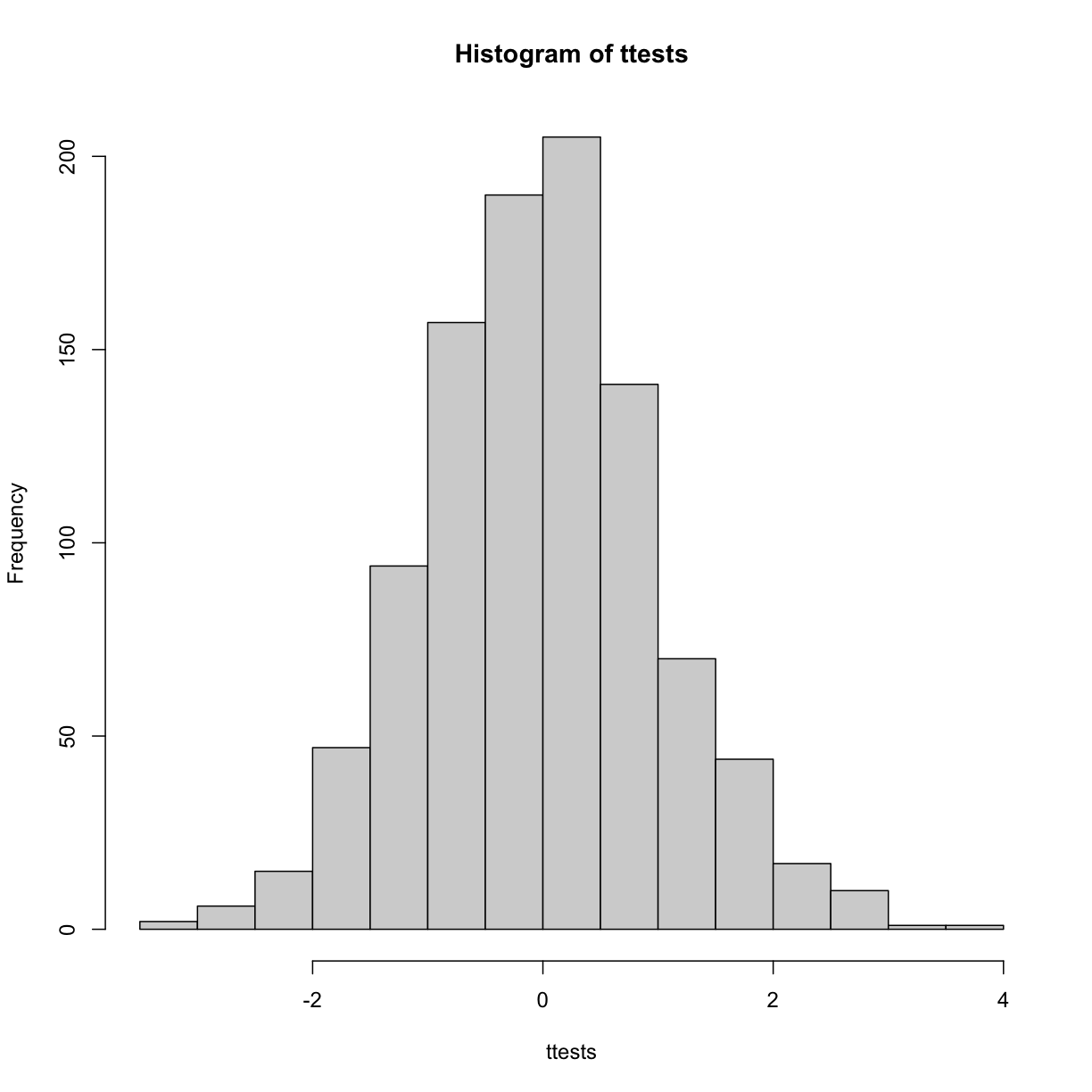So is the distribution of this t-statistic well approximated by the normal distribution? In the next chapter, we will formally introduce quantile-quantile plots, which provide a useful visual inspection of how well one distribution approximates another. As we will explain later, if points fall on the identity line, it means the approximation is a good one.

``````qqnorm(ttests)
abline(0,1)
``````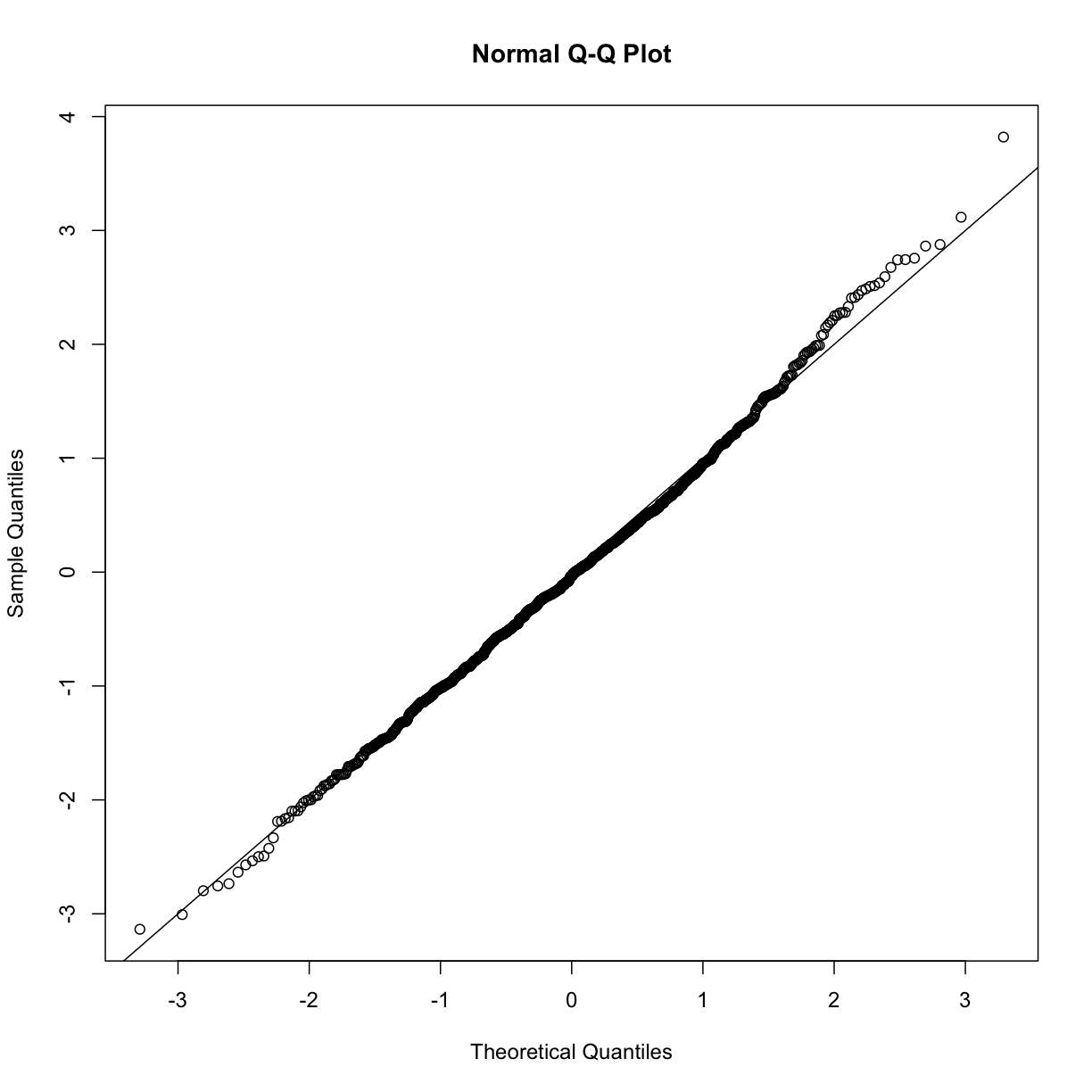This looks like a very good approximation. For this particular population, a sample size of 10 was large enough to use the CLT approximation. How about 3?

``````ttests <- replicate(1000, ttestgenerator(3))
qqnorm(ttests)
abline(0,1)
``````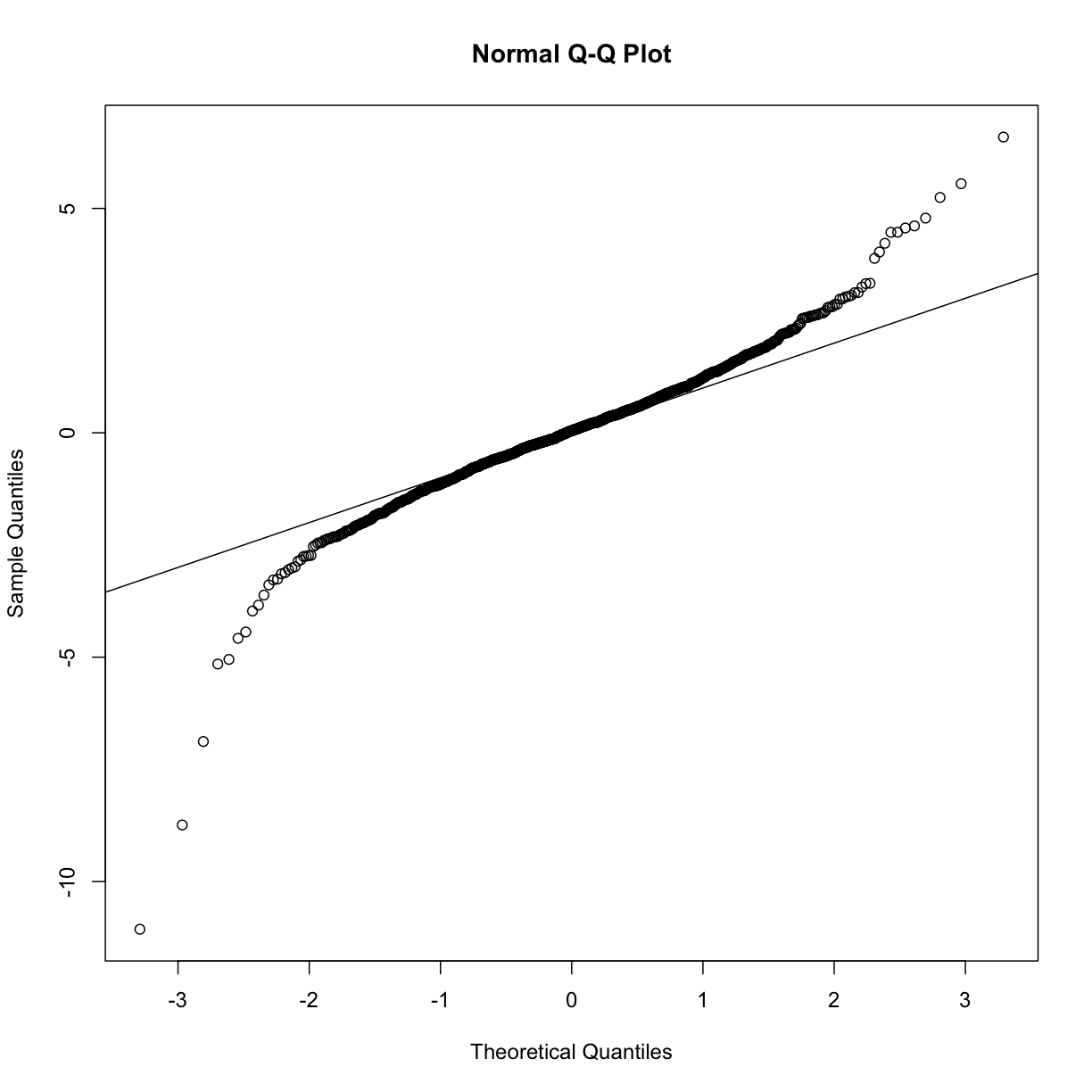Now we see that the large quantiles, referred to by statisticians as the tails, are larger than expected (below the line on the left side of the plot and above the line on the right side of the plot). In the previous module, we explained that when the sample size is not large enough and the population values follow a normal distribution, then the t-distribution is a better approximation. Our simulation results seem to confirm this:

``````ps <- (seq(0, 999) + 0.5)/1000
qqplot(qt(ps, df = 2 * 3 - 2), ttests, xlim=c(-6,6), ylim=c(-6,6))
abline(0,1)
``````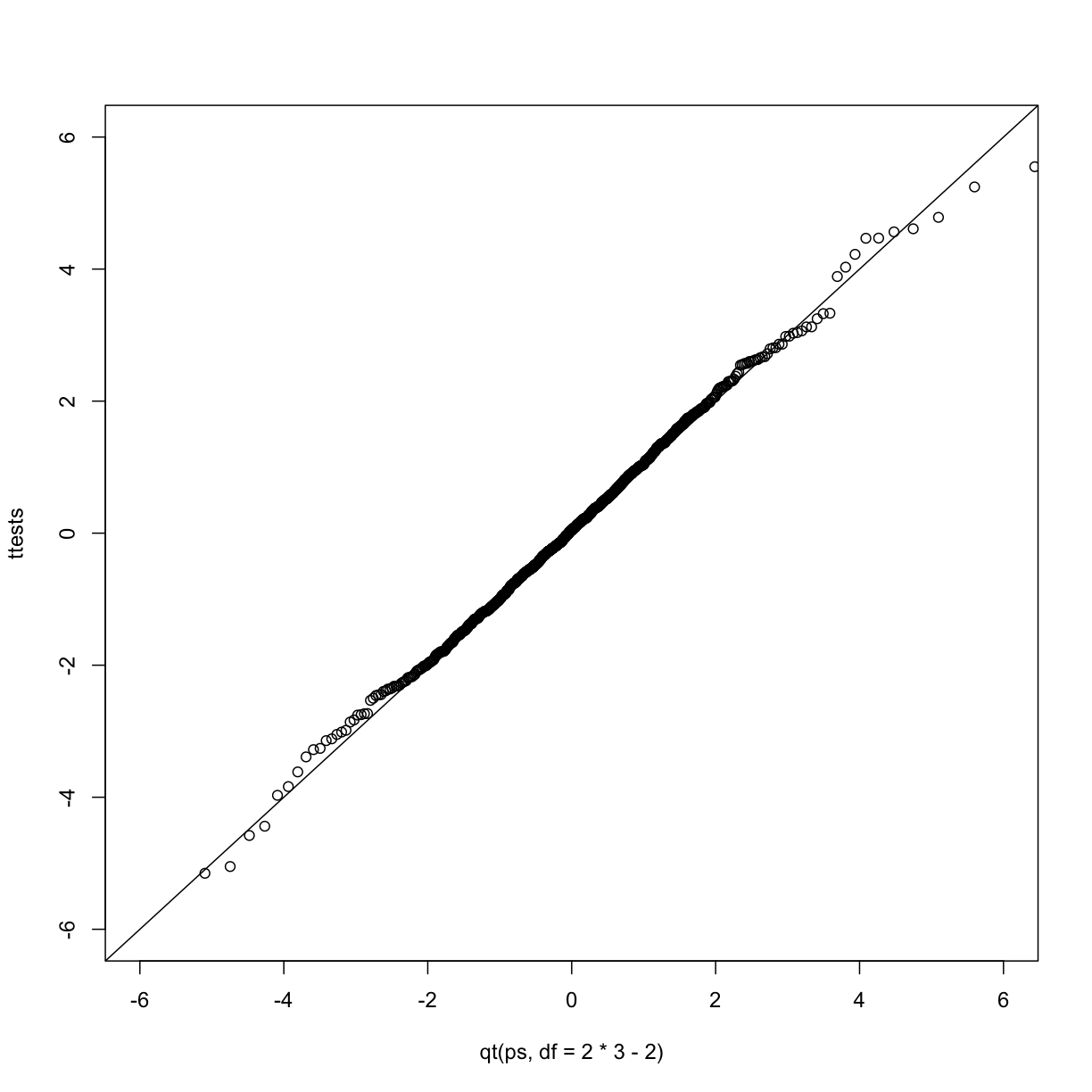The t-distribution is a much better approximation in this case, but it is still not perfect. This is due to the fact that the original data is not that well approximated by the normal distribution.

``````qqnorm(controlPopulation)
qqline(controlPopulation)
``````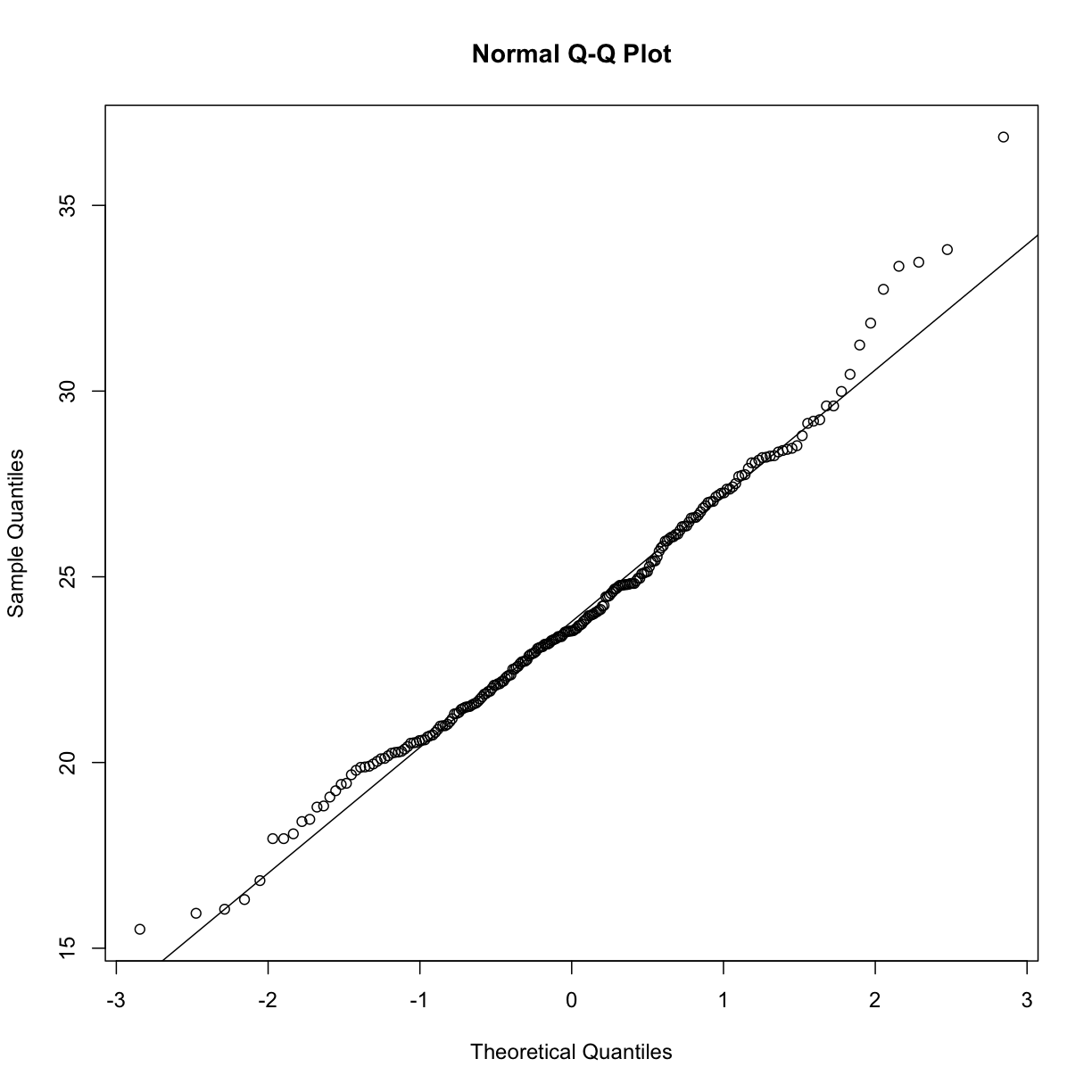## Parametric Simulations for the Observations

The technique we used to motivate random variables and the null distribution was a type of Monte Carlo simulation. We had access to population data and generated samples at random. In practice, we do not have access to the entire population. The reason for using the approach here was for educational purposes. However, when we want to use Monte Carlo simulations in practice, it is much more typical to assume a parametric distribution and generate a population from this, which is called a parametric simulation. This means that we take parameters estimated from the real data (here the mean and the standard deviation), and plug these into a model (here the normal distribution). This is actually the most common form of Monte Carlo simulation.

For the case of weights, we could use our knowledge that mice typically weigh 24 grams with a SD of about 3.5 grams, and that the distribution is approximately normal, to generate population data:

``````controls<- rnorm(5000, mean=24, sd=3.5)
``````

After we generate the data, we can then repeat the exercise above. We no longer have to use the `sample` function since we can re-generate random normal numbers. The `ttestgenerator` function therefore can be written as follows:

``````ttestgenerator <- function(n, mean=24, sd=3.5) {
cases <- rnorm(n, mean, sd)
controls <- rnorm(n, mean, sd)
tstat <- (mean(cases) - mean(controls)) /
sqrt( var(cases)/n + var(controls)/n )
return(tstat)
}
``````

## Exercises

We have used Monte Carlo simulation throughout this chapter to demonstrate statistical concepts; namely, sampling from the population. We mostly applied this to demonstrate the statistical properties related to inference on differences in averages. Here, we will consider examples of how Monte Carlo simulations are used in practice.

1. Imagine you are William Sealy Gosset and have just mathematically derived the distribution of the t-statistic when the sample comes from a normal distribution. Unlike Gosset you have access to computers and can use them to check the results.
Let’s start by creating an outcome. Set the seed at 1, use `rnorm` to generate a random sample of size 5, X1…X5 from a standard normal distribution, then compute the t-statistic t = √5/s with /s the sample standard deviation. What value do you observe?
2. You have just performed a Monte Carlo simulation using `rnorm`, a random number generator for normally distributed data. Gosset’s mathematical calculation tells us that this random variable follows a t-distribution with N-1 degrees of freedom. Monte Carlo simulations can be used to check the theory: we generate many outcomes and compare them to the theoretical result.
Set the seed to 1, generate B= 1000 t-statistics as done in exercise 1.
What percent are larger than 2?
3. The answer to exercise 2 is very similar to the theoretical prediction: 1-pt(2, df=4). We can check several such quantiles using the `qqplot` function. To obtain quantiles for the t-distribution we can generate percentiles from just above 0 to just below 1:B=100; ps = seq(1/(B+1), 1-1/(B+1), len=B) and compute the quantiles with `qt(ps, df=4)`. Now we can use `qqplot` to compare these theoretical quantiles to those obtained in the Monte Carlo simulation. Use Monte Carlo simulation developed for exercise 2 to corroborate that the t-statistic t=pNX/s follows a t-distribution for several values of N.
For which sample sizes does the approximation best work?
A) Larger sample sizes.
B) Smaller sample sizes.
C) The approximations are spot on for all sample sizes.
D) None. We should use CLT instead.
4. Use Monte Carlo simulation to corroborate that the t-statistic comparing two means and obtained with normally distributed (mean 0 and sd) data follows a t-distribution. In this case we will use the `t.test` function with `var.equal=TRUE`. With this argument the degrees of freedom will be df = 2 * N-2 with N the sample size. For which sample sizes does the approximation best work?
A) Larger sample sizes.
B) Smaller sample sizes.
C) The approximations are spot on for all sample sizes.
D) None. We should use CLT instead.
5. Is the following statement true or false?
If instead of generating the sample with `X=rnorm(15)`, we generate it with binary data `X = rbinom(n=15, size=1, prob=0.5)` then the t-statistic `tstat <- sqrt(15) * mean(X)/sd(X)` is approximated by a t-distribution with 14 degrees of freedom.
6. Is the following statement true or false?
If instead of generating the sample with `X = rnorm(N)` with N=500, we generate the data with binary data `X = rbinom(n=500, size=1, prob=0.5)`, then the t-statistic `sqrt(N) * mean(X)/sd(X)` is approximated by a t-distribution with 499 degrees of freedom.
7. We can derive approximation of the distribution of the sample average or the t-statistic theoretically. However, suppose we are interested in the distribution of a statistic for which a theoretical approximation is not immediately obvious. Consider the sample median as an example. Use a Monte Carlo to determine which of the following best approximates the median of a sample taken from normally distributed population with mean 0 and standard deviation 1.
A) Just like for the average, the sample median is approximately normal with mean 0 and SD1/pN.
B) The sample median is not approximately normal.
C) The sample median is t-distributed for small samples and normally distributed for large ones.
D) The sample median is approximately normal with mean 0 and SD larger than 1/pN.

• .

• .

• .

• .